##1. Elementary matrices as projectivities

Since projectivities are represented by matrices, it is reasonable to examine some examples comming from particular matrices. Below is given a series of such matrices together with the description of their action.
Below I examine the effect of elementary symmetric matrices on a projective base and find the kind of the projective map it defines.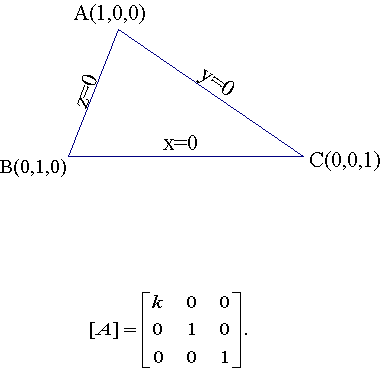All points of the x=0 line (yB+zC) remain fixed. Also point A is fixed under the map F, which is a homology with center at A, axis the line x=0 and homology coefficient 1/k.
Analogous interpretation in the case k takes the place of an other diagonal entry.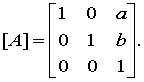All points of the line z=0 remain fixed. All lines through the point O=aA+bB remain invariant.
Hence the map is in this case an elation. To see the invariance of the lines through O, write such a line in parametric form.
Pt = aA+bB + t(uA+vB+wC) = (a+tu)A+(b+tv)B+(twC) and apply on this the transformation giving the line Qt = (a+tu+atw)A+(b+tv+btw)B+twC = a(1+tw)A+b(1+tw)B+t(uA+vB+wC) = (aA+bB)+[t/(1+tw)](uA+vB+wC). Later since the points do not change by multiplying with a (non-zero) constant.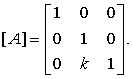All points of the line y=0 remain fixed. All lines through the point C remain invariant. Hence the map is in this case also an elation.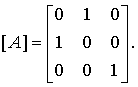A projectivity of period 2, hence a harmonic homology. Fixing the point C and the points D(1,1,1) and E(1,1,0), all on the line x-y=0, which is the axis of the homology. The center is O(1,-1,0).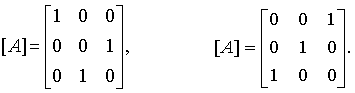Analogous to the previous, the other permutations of rows give harmonic homologies. The axes are correspondingly y-z=0, x-z=0. The centers are correspondingly  O'(0,1,-1) and O''(1,0,-1).

##2. Decomposition of projectivities

The above examples show that all 3x3 elementary matrices represent perspectivities. Since every invertible matrix is a product of such matrices, it follows that every projectivity is a product (composition) of perspectivities. A more geometric aspect of this subject is to be found in the file ProjectivityResolutionPerspectivities.html .
In the file Elation.html I discuss the decomposition of an elation in a product of two harmonic homologies.